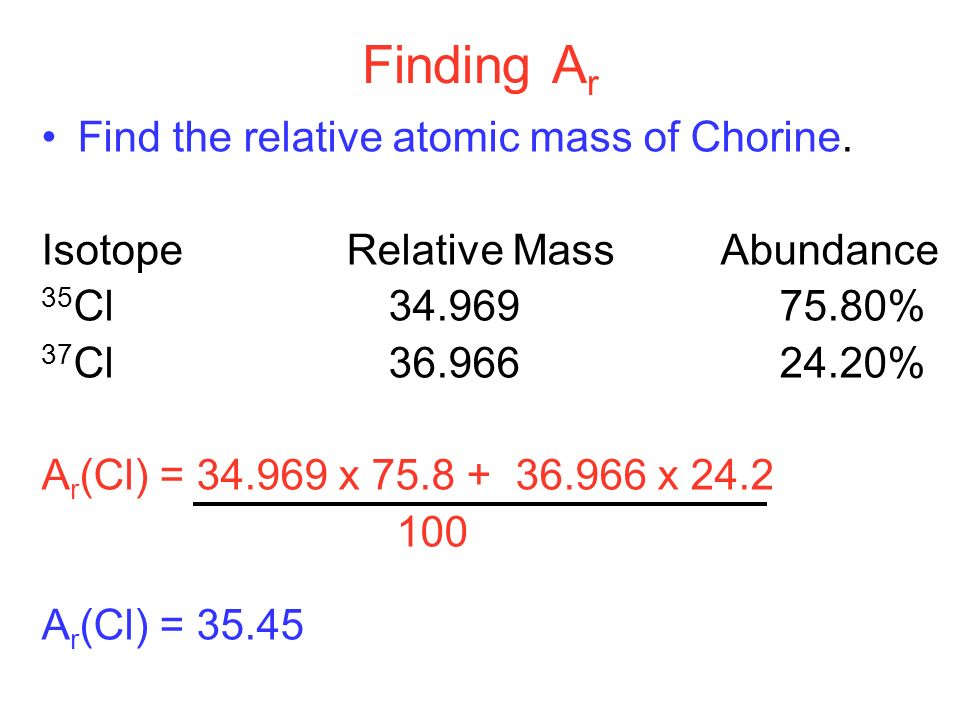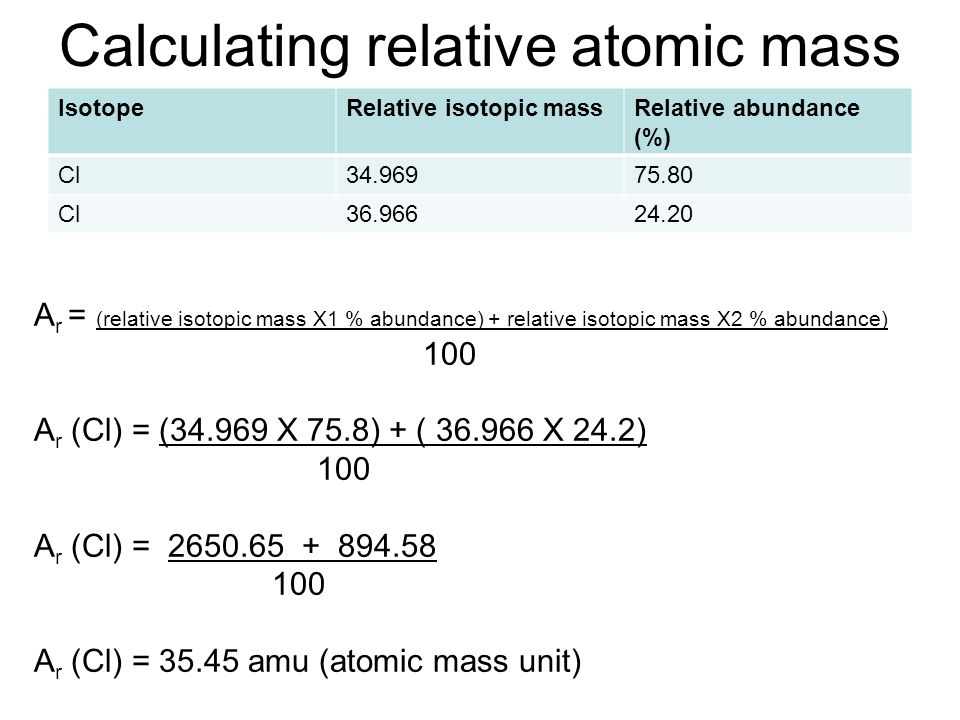# 🤔Way to Find Atomic Mass of Elements🤔

The atomic mass is the mass of the atom of the element. It involves a mass of three subatomic particles which makes one atom: electron, neutron and proton.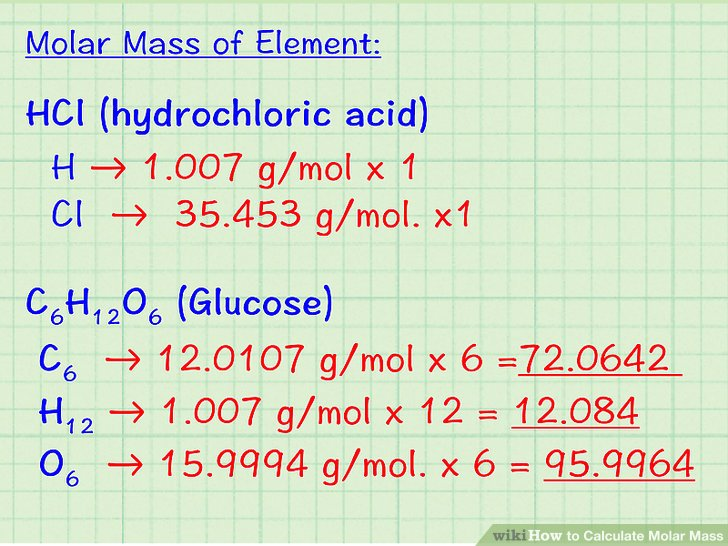Atomic mass is expressed in grams. Since each atom has a very less mass, this is not that helpful. Instead, a mass of an atom is expressed in a Unified atomic mass unit symbol: u. One atomic mass unit can be defined as one-twelfth of the mass of a one carbon-12 atom. 1 u is equal to 1.660538921(73) × 10−27.

### How to Find Atomic Mass

Atomic mass is the total of electron, neutron and proton. There are two ways to find the atomic mass: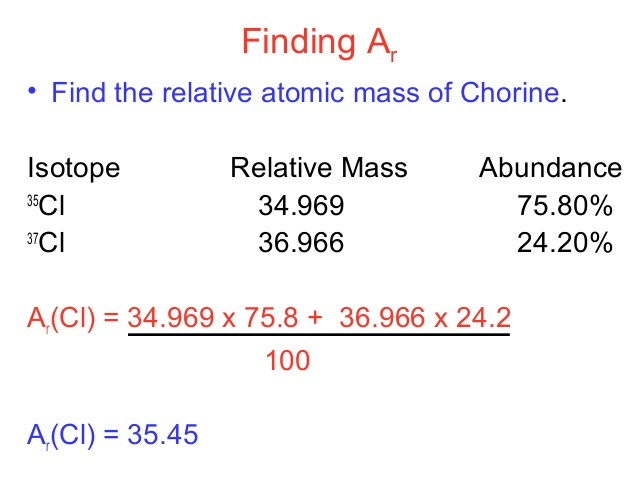1. Finding atomic mass by looking at the periodic table- It can be found by looking at the position of an element in the periodic table.
2. By adding electron, neutron and proton of an element.
3. Average for all atoms of an elements

### How to Calculate Atomic Mass of Isotopes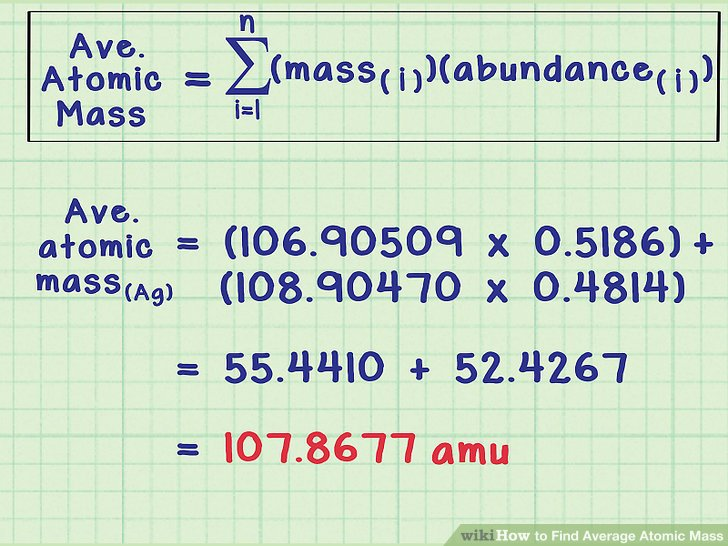The atomic mass of an element is the weighted average of the isotopes of the element. Isotopes are atoms only which have the same atomic number; same no. of the proton but a different number of neutrons.

### Method to Find Average Atomic Mass

The average atomic mass is the sum of the mass of isotopes of the elements; each one is multiplied by its natural abundance.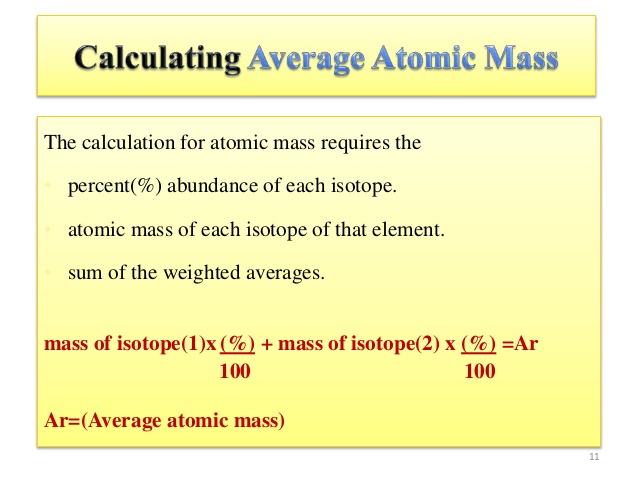f1M1 + f2M2 + … + fnMn is equal to average atomic mass where f stands for the fraction that represents the natural abundance of an isotope and M stands for the mass number of the isotope.

The average atomic mass of the element can be found in the periodic table of elements. When you have the data of the natural abundance of different isotopes of an element, it is easy to calculate the average atomic mass.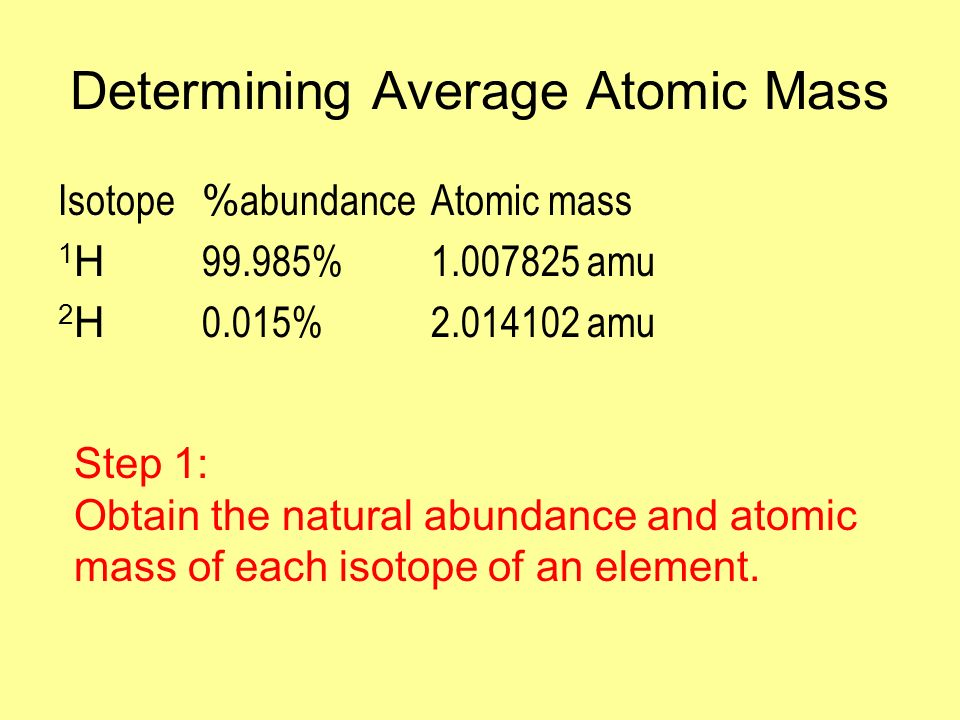In order to calculate the average mass, you have to first convert the percentages into fractions and then calculate the mass numbers. The chlorine isotope which has eighteen neutrons has 0.7577 and a mass number 35 amu. Average atomic mass of chlorine is equal to (0.7577 $\cdot$ 35 amu) + (0.2423 $\cdot$ 37 amu) = 35.48 amu

### How to Find Relative Atomic Mass

• Relative atomic mass is equal to (isotope 1 mass × isotope 1 abundance + isotope 2 mass × isotope 2 abundance + …) ÷ 100
• Relative atomic mass of chlorine is;

Relative atomic mass = (35 × 75 + 37 × 25) ÷ 100

= (2,625 + 925) ÷ 100 = 35.5